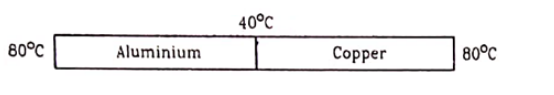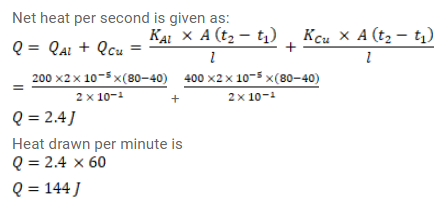# Figure shows an aluminium rod joined to a copper rod.Question:

Figure shows an aluminium rod joined to a copper rod. Each of the rods has a length of $20 \mathrm{~cm}$ and area of cross - section $0.20 \mathrm{~cm}^{2}$. The junction is maintained at a constant temperature $40^{\circ} \mathrm{C}$ and the two ends are maintained at $80^{\circ} \mathrm{C}$ Calculate the amount of heat taken out from the cold junction in one minute after the steady state is reached. The conductivities are $K_{A l}=200 \mathrm{~W} / \mathrm{m}-{ }^{\circ} \mathrm{C}$ and $\mathrm{K}_{\mathrm{cu}}=400 \mathrm{~W} / \mathrm{m}-{ }^{\circ} \mathrm{C}$.Solution: